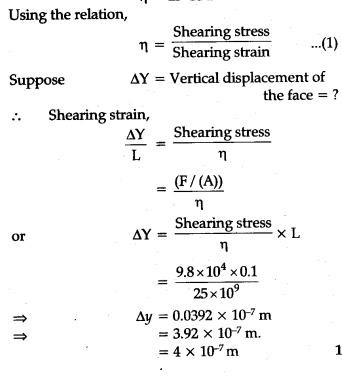# The edge of an aluminium cube is 10 cm long

The edge of an aluminium cube is 10 cm long. One face of the cube is firmly fixed to a vertical wall. A mass of 100 kg is then attached to the opposite face of the cube. The shear modulus of aluminium is 25 G Pa. What is the vertical deflection of this face ?dy=0.0392×10^-7
dy=3.92×10^-7
How is this done